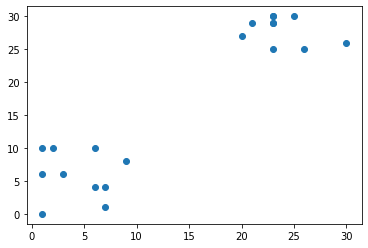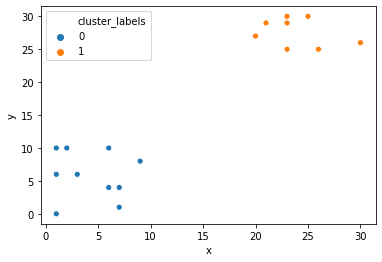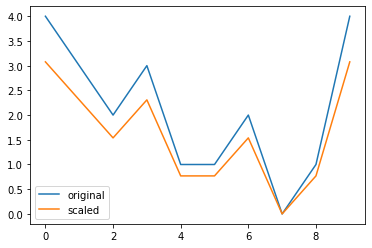import pandas as pd
import numpy as np
import matplotlib.pyplot as plt
import seaborn as sns


## Unsupervised learning: basics

• What is unsupervised learning?
• A group of machine learning algorithm that find patterns in data
• Data for algorithms has not been labeled, classified or characterized
• The objective of the algorithm is to interpret any structure in the data
• Common unsupervised learning algorithms : Clustering, neural network, anomaly detection
• What is clustering?
• The process of grouping items with similar characteristics
• Items in groups similar to each other than in other groups
• Example: distance between points on a 2D plane

### Pokémon sightings

There have been reports of sightings of rare, legendary Pokémon. You have been asked to investigate! Plot the coordinates of sightings to find out where the Pokémon might be.

x = [9, 6, 2, 3, 1, 7, 1, 6, 1, 7, 23, 26, 25, 23, 21, 23, 23, 20, 30, 23]
y = [8, 4, 10, 6, 0, 4, 10, 10, 6, 1, 29, 25, 30, 29, 29, 30, 25, 27, 26, 30]

plt.scatter(x, y)

<matplotlib.collections.PathCollection at 0x15603e78f48>## Basics of cluster analysis

• What is a cluster?
• A group of items with similar characteristics
• Google News: articles where similar words and word associations appear together
• Customer Segments
• Clustering Algorithms
• Hierarchical Clustering
• K-means Clustering
• Other clustering algorithms: DBSCAN, Gaussian Methods

### Pokémon sightings: hierarchical clustering

We are going to continue the investigation into the sightings of legendary Pokémon from the previous exercise. Remember that in the scatter plot of the previous exercise, you identified two areas where Pokémon sightings were dense. This means that the points seem to separate into two clusters. In this exercise, you will form two clusters of the sightings using hierarchical clustering.

df = pd.DataFrame({'x': x, 'y': y})

from scipy.cluster.hierarchy import linkage, fcluster

# Use the linkage() to compute distance

# Generate cluster labels
df['cluster_labels'] = fcluster(Z, 2, criterion='maxclust')

# Plot the points with seaborn
sns.scatterplot(x='x', y='y', hue='cluster_labels', data=df)

<matplotlib.axes._subplots.AxesSubplot at 0x15603ee1948>### Pokémon sightings: k-means clustering

We are going to continue the investigation into the sightings of legendary Pokémon from the previous exercise. Just like the previous exercise, we will use the same example of Pokémon sightings. In this exercise, you will form clusters of the sightings using k-means clustering.

df = df.astype('float')

from scipy.cluster.vq import kmeans, vq

# Compute cluster centers
centroids, _ = kmeans(df, 2)

# Assign cluster labels
df['cluster_labels'], _ = vq(df, centroids)

# Plot the points with seaborn
sns.scatterplot(x='x', y='y', hue='cluster_labels', data=df)

<matplotlib.axes._subplots.AxesSubplot at 0x15604fc6048>## Data preparation for cluster analysis

• Why do we need to prepare data for clustering?
• Variables have incomparable units
• Variables with same units have vastly different scales and variances
• Data in raw form may lead to bias in clustering
• Clusters may be heavily dependent on one variable
• Solution: normalization of individual variables

### Normalize basic list data

Now that you are aware of normalization, let us try to normalize some data. goals_for is a list of goals scored by a football team in their last ten matches. Let us standardize the data using the whiten() function.

from scipy.cluster.vq import whiten

goals_for = [4, 3, 2, 3, 1, 1, 2, 0, 1, 4]

# Use the whiten() function to standardize the data
scaled_data = whiten(goals_for)
print(scaled_data)

[3.07692308 2.30769231 1.53846154 2.30769231 0.76923077 0.76923077
1.53846154 0.         0.76923077 3.07692308]


### Visualize normalized data

After normalizing your data, you can compare the scaled data to the original data to see the difference.

plt.plot(goals_for, label='original')
plt.plot(scaled_data, label='scaled')
plt.legend()
plt.savefig('../images/scaled_data.png')### Normalization of small numbers

In earlier examples, you have normalization of whole numbers. In this exercise, you will look at the treatment of fractional numbers - the change of interest rates in the country of Bangalla over the years.

rate_cuts = [0.0025, 0.001, -0.0005, -0.001, -0.0005, 0.0025, -0.001, -0.0015, -0.001, 0.0005]

# use the whiten() to standardize the data
scaled_data = whiten(rate_cuts)

plt.plot(rate_cuts, label='original')
plt.plot(scaled_data, label='scaled')
plt.legend()

<matplotlib.legend.Legend at 0x1f3a7185788>### FIFA 18: Normalize data

FIFA 18 is a football video game that was released in 2017 for PC and consoles. The dataset that you are about to work on contains data on the 1000 top individual players in the game. You will explore various features of the data as we move ahead in the course. In this exercise, you will work with two columns, eur_wage, the wage of a player in Euros and eur_value, their current transfer market value.

• Preprocess
fifa = pd.read_csv('./dataset/fifa_18_sample_data.csv')
fifa.columns

Index(['ID', 'name', 'full_name', 'club', 'club_logo', 'special', 'age',
'league', 'birth_date', 'height_cm',
...
'prefers_cb', 'prefers_lb', 'prefers_lwb', 'prefers_ls', 'prefers_lf',
'prefers_lam', 'prefers_lcm', 'prefers_ldm', 'prefers_lcb',
'prefers_gk'],
dtype='object', length=185)
fifa['scaled_wage'] = whiten(fifa['eur_wage'])
fifa['scaled_value'] = whiten(fifa['eur_value'])

fifa.plot(x='scaled_wage', y='scaled_value', kind='scatter');

# Check mean and standard deviation of scaled values
print(fifa[['scaled_wage', 'scaled_value']].describe())

       scaled_wage  scaled_value
count  1000.000000   1000.000000
mean      1.119812      1.306272
std       1.000500      1.000500
min       0.000000      0.000000
25%       0.467717      0.730412
50%       0.854794      1.022576
75%       1.407184      1.542995
max       9.112425      8.984064